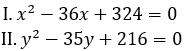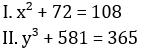Here, we are providing free crash course for LIC AAO Mains as there is left not enough time to deal in details. The questions asked in the quantitative aptitude section are calculative and very time-consuming. But once dealt with proper strategy, speed, and accuracy, this section can get you the maximum marks in the examination. Following is the Quantitative Aptitude quiz to help you practice with the best of latest pattern questions.

Directions (1-5): In each of these questions, two equations numbered I and II are given. You have to solve both the equation and give answer

Q1.if x < y
if x ≤ y
if x > y
if x ≥ y
if x = y or the relationship cannot be established

Q2.if x < y
if x ≤ y
if x > y
if x ≥ y
if x = y or the relationship cannot be established

Q3.if x < y
if x ≤ y
if x > y
if x ≥ y
if x = y or the relationship cannot be established

Q4.if x < y
if x ≤ y
if x > y
if x ≥ y
if x = y or the relationship cannot be established

Q5.if x < y
if x ≤ y
if x > y
if x ≥ y
if x = y or the relationship cannot be established

Directions (6-10): The following questions are accompanied by two statements A and B. You have to determine which statements(s) is/are sufficient/necessary to answer the questions.

Q6. What is the value of rate of interest?
A. A sum of Rs 8000 is invested at simple interest for 3 years in scheme A which offers a certain rate of interest. Amount obtained from scheme A is equal to the amount obtained when Rs 9000 is invested in scheme B for 2 years at C.I.
B. Rate of interest for scheme B is same as rate of interest for scheme A.

Statement A alone is sufficient to answer the question but statement B alone is not sufficient to answer the questions.
Statement B alone is sufficient to answer the question but statement A alone is not sufficient to answer the question.
Both the statements taken together are necessary to answer the questions, but neither of the statements alone is sufficient to answer the question.
Either statement A or statement B by itself is sufficient to answer the question.
Statements A and B taken together are not sufficient to answer the question

Q7. 4 men & 18 women can do a piece of work in 2.5 days, then in how many days 12 woman can complete the same piece of work?
A. Ratio of efficiency of men to women is 3 : 2.
B. 6 men & 6 women can complete the same work in 4 days.

Statement A alone is sufficient to answer the question but statement B alone is not sufficient to answer the questions.
Statement B alone is sufficient to answer the question but statement A alone is not sufficient to answer the question.
Both the statements taken together are necessary to answer the questions, but neither of the statements alone is sufficient to answer the question.
Either statement A or statement B by itself is sufficient to answer the question.
Statements A and B taken together are not sufficient to answer the question

Q8. What will be speed of stream, if speed of boat in still water is 22.5 km/hr?
A. The time taken by boat to cover 120 km upstream is 4 hours more than time taken by boat to cover same distance in downstream.
B. Boat takes total 15 hours to cover 150 km in downstream and upstream.

Statement A alone is sufficient to answer the question but statement B alone is not sufficient to answer the questions.
Statement B alone is sufficient to answer the question but statement A alone is not sufficient to answer the question.
Both the statements taken together are necessary to answer the questions, but neither of the statements alone is sufficient to answer the question.
Either statement A or statement B by itself is sufficient to answer the question.
Statements A and B taken together are not sufficient to answer the question

Q9. What will be sum of two natural numbers X & Y?
A. X & Y both are multiple of 24, while X is 50% more than Y.
B. X/30 & Y/40 both are natural number.

Statement A alone is sufficient to answer the question but statement B alone is not sufficient to answer the questions.
Statement B alone is sufficient to answer the question but statement A alone is not sufficient to answer the question.
Both the statements taken together are necessary to answer the questions, but neither of the statements alone is sufficient to answer the question.
Either statement A or statement B by itself is sufficient to answer the question.
Statements A and B taken together are not sufficient to answer the question

Q10. There is (2a+ 16) students in a class with three streams, i.e. art, science & commerce. The ratio of students who take art to science is 4 : 1. Find total number of students in class.
A. Total students who take art is 8 more than total students who take commerce and probability of selecting one student who take science is 1/8.
B. Total commerce students in class are 25% less than total art students in the class.

Statement A alone is sufficient to answer the question but statement B alone is not sufficient to answer the questions.
Statement B alone is sufficient to answer the question but statement A alone is not sufficient to answer the question.
Both the statements taken together are necessary to answer the questions, but neither of the statements alone is sufficient to answer the question.
Either statement A or statement B by itself is sufficient to answer the question.
Statements A and B taken together are not sufficient to answer the question

Q11. A and B entered in a business by making investment of Rs. 4000 & Rs. 5500 respectively. After six months A & B withdrew Rs. 1000 and Rs. 1500 respectively and C joined them with capital of Rs. 4x. If after one year and three months C received Rs. 2250 as profit share out of total profit of Rs. 12250, then find investment of C?
Rs. 3600
Rs. 3200
Rs. 4400
Rs 3000
Rs. 2800

Q12. Train A can cross a man in 8 sec and a 180 m long platform ‘P’ in 17 sec. If train A cross train B which is running in opposite direction at speed of 108 km/hr in 8 sec, then find time taken by train B to cross platform P?
16 sec
11 sec
14 sec
12 sec
15 sec

Q13. ‘X’ is a bread seller who marked up price of each bread packet by 150% above and allow discounts of 40% and made a profit of Rs. 30 on each bread packet. If ‘X’ allows 37.5% discount on each packet, then find how much profit he gets on selling 80 bread packets?
Rs 2350
Rs 3200
Rs 2500
Rs 2700
Rs 2400

Q14. A sum of money lent at compound interest for 2 years at 20% per annum would fetch Rs. 482 more, if the interest was payable half yearly than if it was payable annually. The sum is :
Rs. 10000
Rs. 20000
Rs. 40000
Rs. 50000
Rs. 25000

Q15. The respective heights and-volumes of a hemisphere and a right circular cylinder are equal then the ratio of their radii is
√2 : √3
√3 : 1
√3 : √2
2 : √3
√2 : 1

You May also like to Read: